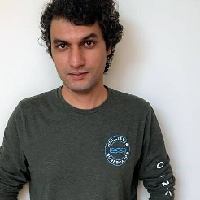TensorFlow (Beginner): Basic Image Classification

Welcome to “Basic Image Classification with TensorFlow”. You will learn to train a neural network to distinguish between images of hand-written digits from 0 to 9. And we will do this with the help of Google’s open source machine learning and deep learning library called TensorFlow. This course will serve as an introduction to both TensorFlow and applied machine learning.Duration (mins)

Learners

5.0 / 5

Rating

We will cover the following tasks in 49 minutes:

Introduction

We will understand the Rhyme interface and our learning environment. You will get a virtual machine, you will need Jupyter Notebook and TensorFlow for this course and both of these are already installed on your virtual machine. Jupyter Notebooks are very popular with Data Science and Machine Learning Engineers as one can write code in cells and use other cells for documentation.

Importing the Libraries

We are going to use Tensorflow’s implementation of Keras - a high level API to build and train the model. In addition to using TensorFlow and Keras, we will also import NumPy and Matplotlib’s PyPlot module which will be used in this example as well. NumPy is the fundamental package for scientific computing with Python. Matplotlib is a Python 2D plotting library which produces publication quality figures in a variety of formats and interactive environments across platforms.

The Dataset

This tutorial uses the MNIST dataset. MNIST consists of a training set of 60,000 examples and a test set of 10,000 examples where each example is a 28x28 grayscale image, associated with a label from 10 classes. The labels are simply numbered from 0 to 9. The images in the dataset are 28x28 NumPy arrays, with pixel values ranging from 0 to 255.

Data Preprocessing

We will scale the input feature values to a range of 0 to 1. This helps Neural Networks learn significantly better. This type of data normalisation is a standard practice when working with Neural Networks. We will need to do this preprocessing to both the training and test data.

Display the Images

We will loop through first 25 images from our training dataset to take a look at how our images look like along with their given labels. We do this with a simple PyPlot figure and then by adding images to its subplot and then displaying them within the Jupyter Notebook.

Building the Model

Neural Networks are built using multiple layers. In this model we have three layers: An input layer, a hidden layer and an output layer. We will use Keras’ Sequential model to create a model with these three layers. For the input layer, we will need to set an input shape. For the hidden layer and the output, we will need to set the number of nodes in those layers and their activation functions.

Compile the Model

Before we can train the model, we need to compile it with some additional settings. We need to specify Loss Function, Optimizer and Metrics. A loss function is an indicator of the difference between predicted values and the actual values. We try to minimize the loss function to steer the model in the right direction during training. Optimizer species how the model is updated based on the input data and the loss function.

Training the Model

Training the neural network model requires a few steps:

• Feed the training data to the model: the `training examples` and `training labels`.
• The model updates its internal parameters and learns to associate the images and labels.
• We ask the model to make predictions on the test data: the `test examples`. Then we verify the results with the `test labels`.

Making Predictions

Predictions for each example gives us an array of 10 numbers. These describe the confidence of the model that the image example belongs to each of the 10 different hand-written digits. To make a definitive prediction, however, we will look at the prediction that the model has the highest confidence value for. Then, one can compare it with the actual label.

More Predictions

We will plot first 25 images with their predictions and their actual labels as given in the test dataset. Correct prediction labels will be in green and incorrect prediction labels will be red to help us make the distinction easily. We will use the same method for plotting these images as before: by first creating a PyPlot figure and then iterating over images as we add them to a subplot.

Watch Preview

Preview the instructions that you will follow along in a hands-on session in your browser.I am a Software Engineer with many years of experience in writing commercial software. My current areas of interest include computer vision and sequence modelling for automated signal processing using deep learning as well as developing chatbots.

How is this different from YouTube, PluralSight, Udemy, etc.?
In Rhyme, all projects are completely hands-on. You don't just passively watch someone else. You use the software directly while following the host's (Amit) instructions. Using the software is the only way to achieve mastery. With the "Live Guide" option, you can ask for help and get immediate response.